# Understanding Pointwise Mutual Information in Statistics

Contents

### Intro

The term mutual information is drawn from the field of information theory. Information theory is busy with the quantification of information. For example, a central concept in this field is entropy, which we have discussed before.

If you google the term “mutual information” you will land at some page which if you understand it, there would probably be no need for you to google it in the first place. For example:

Not limited to real-valued random variables and linear dependence like the correlation coefficient, mutual information (MI) is more general and determines how different the joint distribution of the pair (X,Y) is to the product of the marginal distributions of X and Y. MI is the expected value of the pointwise mutual information (PMI).

which makes sense at first read only for those who don’t need to read it. It’s the main motivation for this post: to provide a clear intuition behind the pointwise mutual information term and equations, for everyone. At the end of this page, you would understand what mutual information metric actually measures, and how you should interpret it. We start with the easier concept of conditional probability and work our way through to the concept of pointwise mutual information.

### Conditional probability

If you know the result of a fair, six-sided die is larger than 4, the probability that it is a 5 is 1/2- while if you don’t know the result is larger than 4, then the probability remains 1/6. So the fact that you know the result is larger than 4 made a big difference for you in this case. But how big of a difference? We want to quantify how big is this difference compared to say, you know that the result of the die roll is larger than 2, or don’t know anything for that matter.

In the example above we implicitly used the conditional probability formula: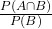with A being the event “larger than 4”,being the event “result is equal to 5”, andmeans both A and B occurred simultaneously.

### Pointwise mutual information

Those “events” above are just random variables: what can happen? what is the probability of each of the possible outcomes? If we denote those random variables as x and y, the formula for pointwise information is very closely related to that of conditional probability. The link between conditional probability and mutual information is your main engine for understanding this topic. The formula for pointwise information isForget about the log operator for a second. Let’s massage this formula:Let’s focus on the last expression. As you can see, it’s the conditional probability of X given Y times. If Y and X are independent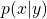equals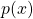, and in that case you would have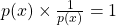and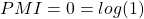.

How “important” is the event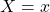? ifthen the event X=x is not really important is it? think a die which always rolls the same number; there is no point to consider it. But, If the eventis fairly rare → p(x) is relatively low →is relatively high → the value of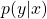becomes much more important in terms of information. So that is the first observation regarding the PMI formula.

### A practical example and some additional intuition

In this code we pull some ETF data from yahoo, for TLT (US treasury bonds) and SPY (US S&P 500 stocks). We create two series of daily returns for those two tickers.

Now let’s define the our random variables. X would be: “returns of TLT is below it’s 5% quantile”. The random variable Y would be: “returns of SPY is below it’s 5% quantile”, so two binomial random variables.

Now, based on the pointwise mutual information formula we compute the PMI measure:

The PMI measure is about -1.65. What (the hell) does that mean?

Pointwise mutual information measure is not confined to the [0,1] range. So here we explain how to interpret a zero, a positive or, as it is in our case, a negative number. The case where PMI=0 is trivial. It occurs for log(1) =0 and it means thatwhich tells us that x and y are independents. If the number is positive it means that the two events co-occuring in a frequency higher than what we would expect if they would be independent event. Why? because(or equivalently) is larger than 1 (if it’s smaller than 1, the log is negative). In our case the number is lower than one, meaningwhich means we see more of X=x than we see y given that X=x.

Let’s talk numbers to make it more tangible. The individual probabilities are p_x = p_y = roughly 5% (by construction here). If the events\variables are independent we would expect to see both occur simultaneously around 0.05^2 = 0.25% of the time. Instead we see those events co-occur only
> p_xy *100  0.07952286
so only 0.08% of the time. So we see this joint event roughly one third of what we would expect relatively to the events being independent (approximately 0.08/0.25). This 0.316 figure is what continues into the log operator and produces us with the negative number.
(p_ab/p_b)/p_a  0.3167045

log2((p_ab/p_b)/p_a)  -1.658791
Practically it means that the number of times where both stocks and bonds are having a bad day (being below their 5% quantile), is much lower compared to them having bad days individually. So seeing a bad day for one of those does not drag a bad day for the other, on the contrary. Which makes sense given the bonds-as-a-hedge-againt-stock-market-doom textbook argument.

### Summary

The pointwise mutual information can be understood as a scaled conditional probability.

The pointwise mutual information represents a quantified measure for how much more- or less likely we are to see the two events co-occur, given their individual probabilities, and relative to the case where the two are completely independent.Information Theory: A Tutorial Introduction

### 6 comments on “Understanding Pointwise Mutual Information in Statistics”

1.Dorian says:

When you say: “If Y and X are independent, there is no meaning to the multiplication (it’s going to be zero times something).”

Do you actually mean “If Y and X are mutually exclusive” ?

1.Eran Raviv says:

I don’t

2.Abbas says:

In the section: Pointwise mutual information your chain rule formula has a tiny mistake:
P(x,y)/P(x) = P(y/x) and is not equal to P(x/y)

3.Andrea says:

Thank you for the very helpful explanation. Two corrections:

+1 to Abbas’s comment. P(y|x) and P(x|y) should be swapped in the section where you work out the PMI formula.

1.Eran Raviv says:

Andrea, Dorian and Abbas,
Now updated. Sincere thanks.
Eran